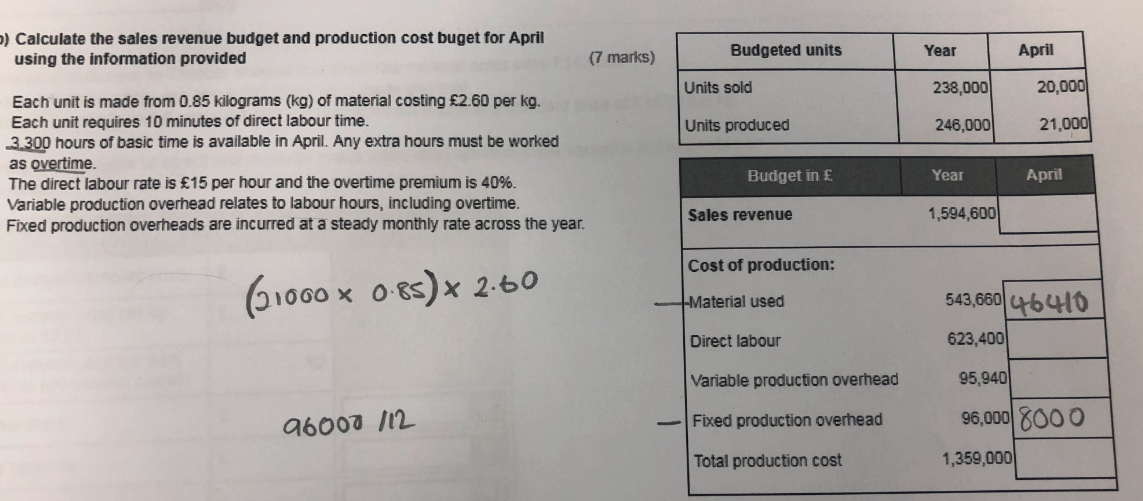# Sales Revenue

Hi,

can someone help me on the below I've worked out someone of the answers but the other i cannot work out.

thanks
Sophie

•• Sales revenue in April - (£1,594,600/238,000) * 20,000 = £134,000

Direct labour
Total labour hours in April - 21,000/6 = 3500
Total hours worked overtime = 3500-3300 = 200. Overtime rate = 15*1.4 = £21.00 per hour.
Direct labour cost - (3300*15) + (200*21) = £53,700.

Total hours for year - 246,000/6 = 41,000 hours
Variable production overhead per hour - 95,940/41,000 = £2.34
Variable overhead production overheads in April - £2.34 * 3500 = £8,190.
Matthew Dearlove MAAT
Currently on CIMA Management Level. Passed P2, F2 and E2. Preparing for November 19 MCS.
• Sales revenue in April - (£1,594,600/238,000) * 20,000 = £134,000

Direct labour
Total labour hours in April - 21,000/6 = 3500
Total hours worked overtime = 3500-3300 = 200. Overtime rate = 15*1.4 = £21.00 per hour.
Direct labour cost - (3300*15) + (200*21) = £53,700.

thanks so much that makes sense now